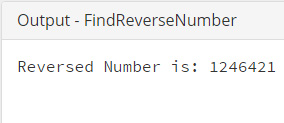## Java Programming Examples Tutorial Index

This Java program is used to find reverse number.

Example:

``````public class FindReverseNumber {

public static void main(String[] args) {

//number defined
int number = 1234;
int reversedNumber = 0;
int temp = 0;

while (number > 0) {
//modulus operator used to strip off the last digit
temp = number % 10;

//create reversed number
reversedNumber = reversedNumber * 10 + temp;
number = number / 10;
}

//output
System.out.println("Reversed Number is: " + reversedNumber);
}
}``````

Program Output:Explanation:

This is a Java program which is used to find the reverse of a number. So in this program you have to first create a class name FindReverseNumber and within this class you will declare the main() method. Now inside the main() you will take two integer type variables. One will be initialized with the value whose reverse is to be done. Secondly, another variable has been defined reverse number which will store the value after the reverse operation is done.

Also you have to take one temporary variable for storing integer value within the calculation. Now you have to use a looping statement, here while loop has been used. Inside that while loop the condition is being checked whether taken is greater than zero or not. If the condition becomes true the following statements gets executed:

temp = number%10;

reversedNumber = reversedNumber * 10 + temp;

number = number/10;

first statement finds the modulus of the number taken and store it in a temporary variable. The next statement is used to initialize the calculation (multiplication) of reverseNumber with 10 and add it with temp and then storing it back to reverseNumber variable. And the final statement number is divided by 10 and is getting stored on the variable itself.

And lastly, the System.out.println("Reversed Number is: " + reversedNumber); statement gets executed, printing the value of reverse number.